# HOUR Function

Categorized as a Date and Time function that returns the hour component of the time

It's amazing how Amazon fulfills its customers' orders exactly on time. Everything from ordering the product to its delivery depends on the timing. If the timing is wrong, the customer can potentially face a delay in the product's delivery.How Amazon manages its warehouse supplies and delivers the products to its customers is intricate. For example, if we say it takes one day for a product to reach the nearest warehouse and two hours to make the delivery, we can use the HOUR function to club all similar products into one list.

The function is categorized as a Date and Time function that returns the hour component of the time between 0 (12:00 A.M.) and 23 (11:59 P.M.), depending on the time value used as the input.Returning to our Amazon example, suppose that 100 products are ordered from NYC between 9 - 10 P.M. on the 4th of April 2022. Using this function, all these deliveries could be clubbed together with their expected delivery time at 9:00 P.M. the next day.

If the delivery time is 9:00 P.M., all 100 products must reach the warehouse by 7:00 P.M.

By maintaining a strict schedule for the supply chain, potential losses could be avoided, and customer satisfaction could be improved - all with a simple time function such as HOUR.

## The syntax for the HOUR function

The syntax for the function is:

=HOUR(serial_number)

where,

serial_number = (required) the time value from which we want to extract the hour component.

This is a required argument that even accepts time as text strings (for example, "10:30 A.M.") or decimal values (such as 0.84375, which corresponds to 8:15 P.M.).

Example

To understand the function better, we will see different instances of time from which you can extract the hour component.

Illustrated below are various formats in which you will usually encounter the time in Excel.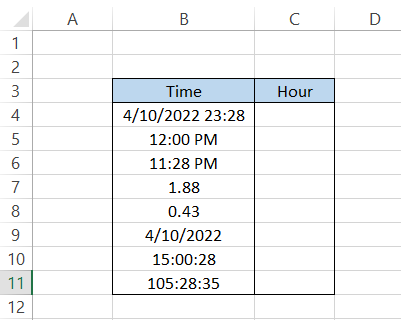To find the hour component for each value in column B, use the formula =HOUR(B4) and drag it down up to the last cell, which will give you the result: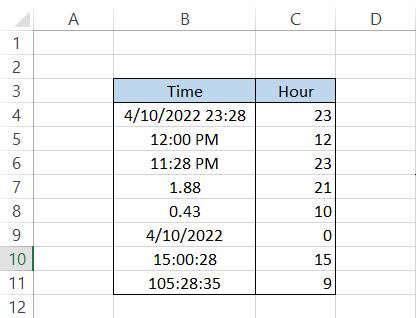Interpretation

• For date and time 4/10/2022 23:28, the function ignores the date and minutes and only returns the hour in cell C4, which is 23.
• For time 12:00 P.M., the function returns 12.
• For time 11:28 P.M., the function returns the result as 23. Even if the hour in our time is 11, remember that the number is extracted based on the 24-hour clock (from 0-23).
• Well, this is something new. By using the HOUR function on 1.88, we get the result of 21. Let's interpret 1 as a 24 hour period i.e 1 = 24 hours. But the value we have is more than 1, meaning the time is more than 24 hours.

If we change the format of 1.88 to [h]:mm: ss, we see that the time we get is 45:07:12.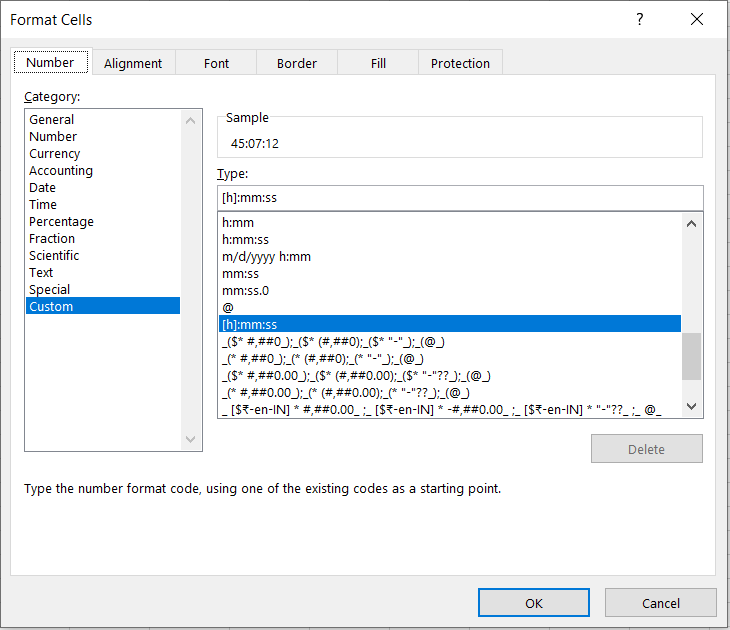But we aren't getting the result as 45 as well. The thing is, once the 23:59 is completed, the clock resets to zero again.

By subtracting 24:00:00 from 45:07:12, we get the result as 21:07:12, the same result for our hour component.

• Again, a similar time but now it's less than 24 hours (the value for 24 hours is 1). For this, we get 10. You can always cross-check by changing the format of the value to [h]:mm: ss by using Ctrl + 1 key on your keyboard
• Next, we only have the date 04/10/2022 in cell B9. If we do not have any specified time, Excel assumes a default time of 12:00 A.M.

As we already know, Excel returns only the numbers from 0-23 from 12:00 A.M. to 11:59 P.M. time, so the result we get in Excel is zero.

• When the time is represented in HH:MM: SS format, such as 15:00:28, the HOUR function ignores the minutes and seconds to only return the value as 15.
• This is similar to what we saw earlier; if the time is 105:28:35, we need to subtract multiples of 24:00:00 to get the present time. The time 105:28:35 could also be interpreted as 4 days, 9 hours, 28 minutes, and 35 seconds.

Thus, the function extracts the 9, our result in cell C11.

## Practical Example #1

Assume that you day trade for a living. You trade your favorite stocks, MicrosoftNetflix, and Tesla. You prepare an Excel sheet to record all your transactions so you can better understand how profitable you have been.

The hypothetical data is illustrated below: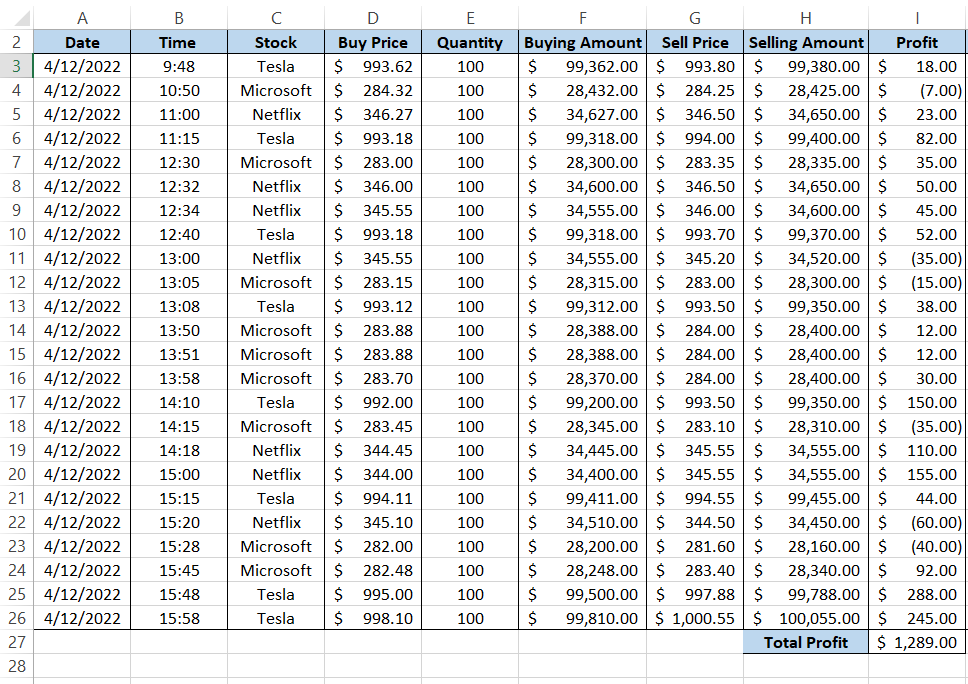Let's say you want to know how much profit you have earned each hour from the start of the day. For this, you will use the formula as =HOUR(B3) in column J.

This will give you the result as illustrated below: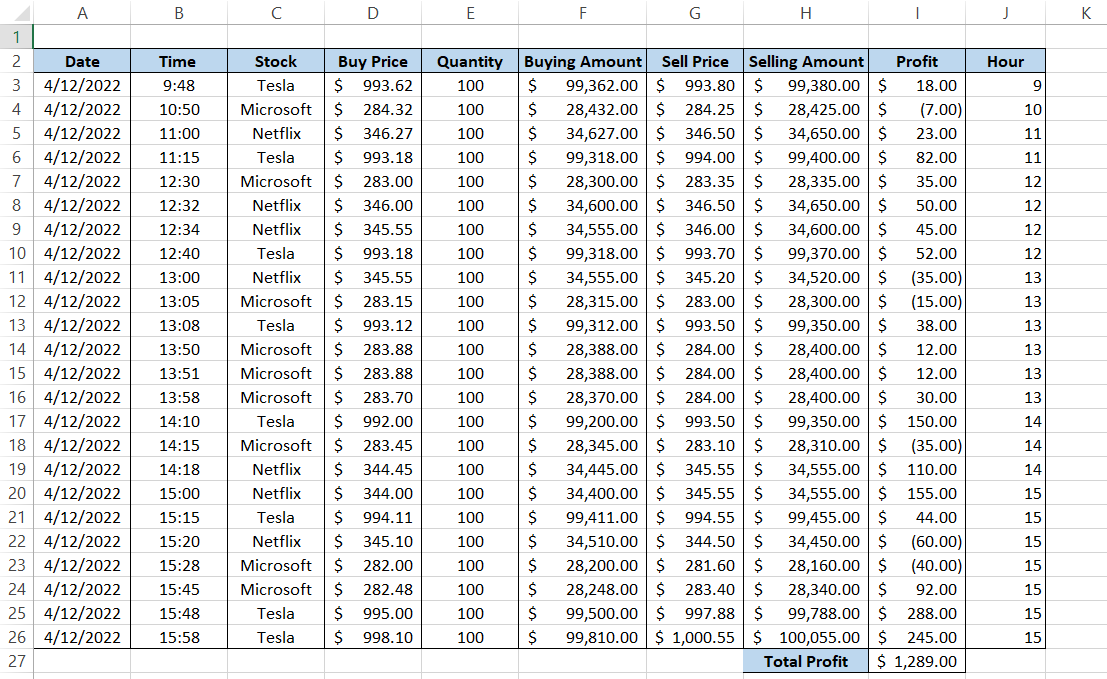Now, how do we represent the recurring data in a unique format? Pivots! Nothing beats pivots when you are working on data analysis and need to remove the duplicates, like in the scenario above.
We will only add the 'Profit' and 'Hour' to the Pivot Table field area. This will return the following table: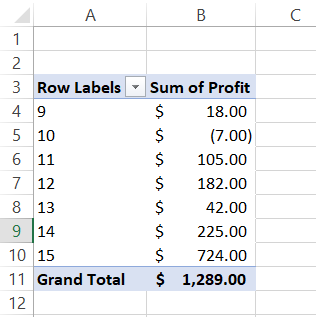This makes our analysis of profit very simple. As you can see, the most profit was earned during the closing stages of the market, between 2 P.M. and 4 P.M.

## Practical Example #2

One of the applications where the rules of the function are applied is the biometric fingerprint attendance system.

Assume that at the end of the month, you receive the sheet for all the employee ins and outs and need to check whether the employees have completed the necessary working hours in the office (just an example! We hope the working conditions should never be so severe that it takes the fun out of work).

The data is illustrated below: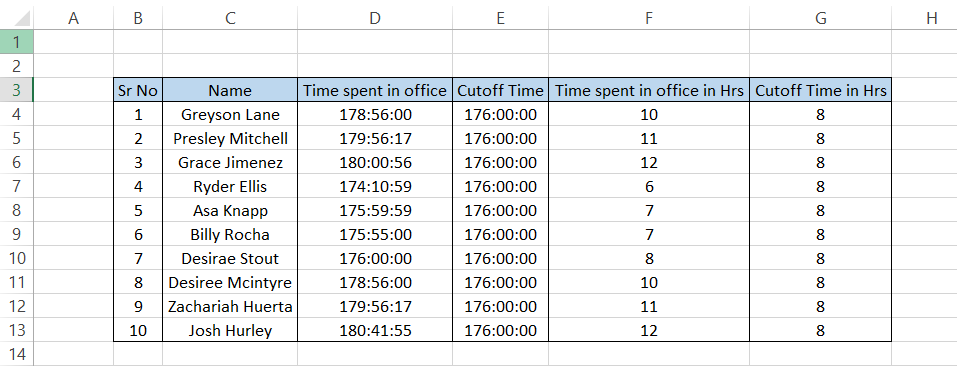The column' Time spent in the office in Hrs' represents how many hours the employees worked extra or fell short. In columns F and G, we used the formula =HOUR(D4) and =HOUR(E4), respectively.

So, remember, the next time you walk out that door early, you are being watched!

Important things to remember:

• If you do not input a valid Excel time as the serial_number argument, the function will give you the #VALUE! Error. Decimal values are also excluded from the errors, where the range of 24-hour clock is represented between 0 and 1.### Everything You Need To Master Excel Modeling

To Help You Thrive in the Most Prestigious Jobs on Wall Street.

Researched and Authored by Akash Bagul | Linkedin

Reviewed and edited by James Fazeli-Sinaki | LinkedIn